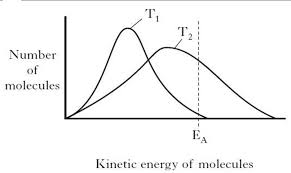## How to Calculate and Solve for Mass, Velocity and Kinetic Energy | The Calculator EncyclopediaThe image above represents kinetic energy.

To compute for the kinetic energy, two essential parameters are needed and these parameters are mass (m) and velocity (v).

The formula for calculating the kinetic energy:

K.E. = 0.5mv²

Where;
K.E. = Kinetic Energy
m = Mass
v = Velocity

Let’s solve an example;
Find the kinetic energy when the mass is 6 and the velocity is 18.

This implies that;
m = Mass = 6
v = Velocity = 18

K.E. = 0.5mv²
K.E. = 0.5[6 x 18²]
K.E. = 0.5[6 x 324]
K.E. = 0.5
K.E. = 972

Therefore, the kinetic energy is 972 Joules (J).

Calculating the Mass when Kinetic Energy and Velocity is Given.

m = K.E / 0.5v2

Where;
m = Mass
K.E. = Kinetic Energy
v = Velocity

Let’s solve an example;
Find the mass when the kinetic energy is 320 and a velocity of 20.

This implies that;
K.E. = Kinetic Energy = 320
v = Velocity = 20

m = K.E / 0.5v2
m = 320 / 0.5 x 202
m = 320 / 0.5 x 40
m = 320 / 20
m = 16

Therefore, the mass is 16 kg.